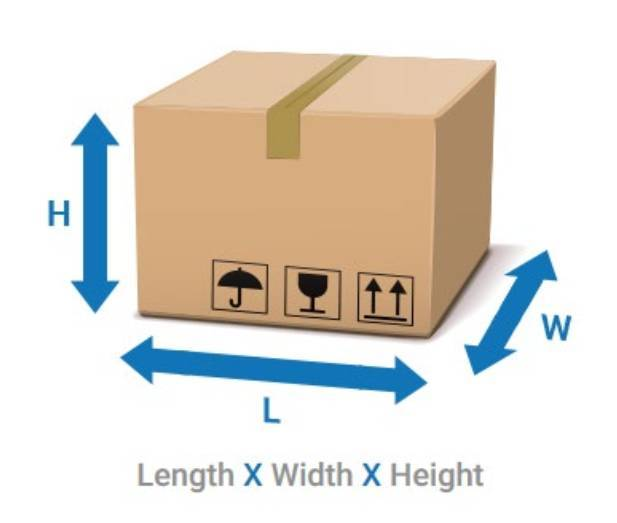## WEIGHT CALCULATOR

### Units of Measure

CM/KG length*width*height/5000 Inches/Pounds length*width*height/139 The Volumetric Tool rounds each dimension (length, width and height) to the nearest whole number using standard rounding rules. The resulting dimensional weight is then rounded to the next full pound or half kilogram. For example, a box with dimensions of 30.4”(L) x 19.6”(W) x 19.5”(H), will be considered to be 30”(L) x 20”(W) x 20”(H) = 12,000 cubic inches, and will be divided by 139 = 86.3 lbs. for a “Dimensional Weight” of 87 lbs

#### Units of Measure:### Air freight volumetric (chargeable weight) calculator

Air freight calculator is based on volume measured weight also known as volumetric weight. The air freight will always be charged per kilogram against the greater weight whether it is the actual or volumetric weight.

#### Choose metric system:

Air freight chargeable weight calculation explained

• 6000 cubic centimeters per kg.
• 366 cubic inches per kg.
• 166 cubic inches per lbs (pound).

Example: Volumetric calculation

Example: Volumetric calculation 1 pallet: 140 x 100 x 90 cm Actual Volume - 1.4 x 1.0 x 0.9 = 1.26 cubic meters Calculating Volumetric Weight 1.26 Cbm Divide / by 0.006 = 210 – This will become the volumetric weight of the shipment in Kilograms.

The actual weight of the pallet may be 150 kgs from which the Airfreight will be charged against the volumetric weight 210 kgs. If the pallets actual weight is 260 Kgs it would be charged against the actual weight of the shipment.

Scroll to Top